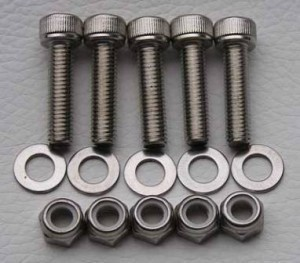•• # Bolt Dimensions

Before moving on to bolt sizes, it should be stated that there is no clear distinction between a bolt and screw.There have been some attempts to define each one, but for practical purposes it is not important. Both bolts and screws are considered fasteners.

### Common Bolt Dimensions

Bolt dimensions are measured in terms of grade mark, nominal size range (in inches) and their mechanical properties. These properties are the proof load (psi), yield strength min (psi) and tensile strength (psi).

The fastener with a specification of SAE J429 is composed of medium carbon steel and has a nominal size range of 1/4 thru 1-1/2. The proof load is 33,000 and the yield 36,000. The tensile strength is 60,000.

The ASM A307 Grades A&B are composed of low carbon steel. This bolt size has a nominal size of ¼ thru 4 and has a tensile strength of 60,000. The B8 ASTM A193 Grade B8 uses AISI 304 and has a nominal size of ¼ thru ¾. The yield strength is 100,000 and the tensile strength is 80,000.

The ASTM A320 Grade L7 has a yield strength of 105,000 and a tensile strength of 125,000. The ASTM A320 Grade B8T has a nominal size of ¼ and bigger. The yield is 30,000 and the
tensile is 75,000.

The SAE J429 Grade 5 has a nominal size of ¼ thru 1 and over 1 to ½. The proof load is 85,000 and the yield is 92,000. The tensile strength is set at 120,000.

### Other Bolt Sizes

The ASTM A449 has the following dimensions: ¼ thru 1 (nominal size) and proof load of 85,000. The yield is 92,000 and the tensile strength is 120,000. The SAE J429 Grade 5.2 has a size of ¼ thru. The proof load, yield and tensile are 85,000, 92,000 and 120,000 respectively.

The ASTM A325 Type has the following specifications: ½ thru to 1 with a proof load of 85,000, yield of 92,000 and tensile of 120,000. The ASTM A490 has a size of ½ thru 1 ½. The proof load is 120,000 and the yield is 130,000. The tensile is 170,000.

### How to Find the Right Bolt Size

The key is the thread. These are the ridges that surround the bolt. If the thread is too big the bolt won’t fasten at all. If it’s too small, it won’t fit in. When looking for bolts, look for signs like M5 or something similar. The M stands for millimeter and the 5 is its size. So an M5 is a 5 millimeter bolt. Depending on where you live, different measurements may be used.

There are other types of bolts you’ll come across in hardware stores. The breakaway bolt has a hollow threaded shank. These are utilized in fire hydrants and in some airplanes. The tap bolt has threads all the way up to the head, while the toggle bolt comes with a nut referred to as the wing.

Understanding of the various bolt sizes and their properties are the keys to utilizing them well. It really isn’t as complicated as it seems and it will only take a few uses for you to get familiar with them.